Next: 5.6 Contact Voltage Variable Up: 5. Contacts and Boundaries Previous: 5.4.2 Dirichlet Type

# 5.5 Contact Model

The generalized boundary condition is the constitutive relation for the contact potential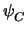and reads

 f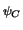=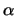.+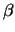. IC +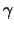. QC -= 0 (5.20)

where QC is the contact charge and IC = InC + IpC +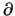QC/t the contact current. It should be noted that all these quantities are solution variables, which simplifies the formulation of the contact models.

For the special case of a traditional voltage controlled contact= 1,== 0, and= V0 and (5.20) degenerates to

 f=- V0 = 0 (5.21)

Modeling a series contact resistance using= RC one gets

 f=+ RC . IC - V0 = 0 (5.22)

For a current controlled contact= 1,== 0, and= I0 and (5.20) degenerates to

 f= IC - I0 = 0 (5.23)

For a charge controlled contact== 0,= 1, and= Q0 and (5.20) degenerates to

 f= QC - Q0 = 0 (5.24)

Using different units for the coefficients,,, and(5.20) can be interpreted in different ways. These include a parallel conductance and capacitance to ground for a current controlled contact, and a series resistance and parallel capacitance to ground for a voltage controlled contact.

As (5.20) is normally not diagonal-dominant it is pre-eliminated. In (5.23) the main-diagonal entry is zero, therefore fIC must be eliminated first in order to get the derivatives with respect to.Next: 5.6 Contact Voltage Variable Up: 5. Contacts and Boundaries Previous: 5.4.2 Dirichlet Type
Tibor Grasser
1999-05-31